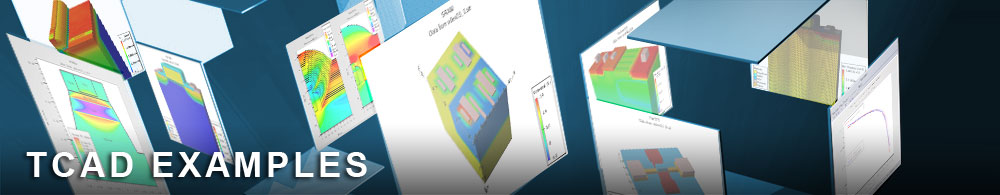Inductance Q Factor Optimization Using LM Algorithm

## vwfex11 : Inductance Q Factor Optimization Using LM Algorithm

Requires: VWF, QUEST

This example demonstrates how to use the optimization feature in VWF to fit a curve (i.e Q factor as function of the frequency). In this example, in comparion to vwfex10.in, a Levenberg-Marquardt algorithm is used. In this case The targetted curve is directly imported in VWF in a TonyPlot user data format, whereas the simulated one is the result of an extract statement.

extract name="Q" curve (da.value."Frequency",da.value."Q_1") outfile="extract.dat"

To open and run this example, select the Help Load example button in the VWF explorer. This will import all examples into your database. Once imported, the examples will be available under the directory called examples.